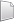# HS-1015: Work with an Internal Math Model

Learn how to set up a study on simple functions defined using an Internal Math model.

In this tutorial you will set up a beverage can design of experiments to see how design variables effect the responses. The beverage has the following design variables and output responses:
Design variables
Diameter
Height
Output responses
Material Cost
Volume

## Perform the Study Setup

1. Start HyperStudy.
2. Start a new study in the following ways:
• From the menu bar, click File > New.
• On the ribbon, click.
3. In the Add Study dialog, enter a study name, select a location for the study, and click OK.
4. Go to the Define Models step.
5. Add an Internal Math model.
2. In the Add dialog, select Internal Math and click OK.
6. Go to the Define Input Variables step.
7. Create two input variables.
1. Click Add Input Variables twice.
2. In the work area, Label column, change the labels for the two input variables to Diameter and Height.
3. Change both input variable's lower, nominal, and upper bounds to the values indicated in Figure 1.

## Perform Nominal Run

1. Go to the Test Models step.
2. Click Run Definition.
An approaches/setup_1-def/ directory is created inside the study Directory. The approaches/setup_1-def/run__00001/m_1 directory contains the input file, which is the result of the nominal run.

## Create and Evaluate Output Responses

In this step you will create the output responses, Cost and Volume.

1. Go to the Define Output Responses step.
2. Create two output responses.
1. Click Add Output Responses two times.
2. In the work area, Label column, change the labels for the output responses to Cost and Volume.
3. In the Expression column, enter the following:
1. For Cost, enter 2*(pi*var_1^2/4)+var_2*pi*var_1.
2. For volume, enter (pi*var_1^2/4)*var_2.
4. Click Evaluate to extract the response values.